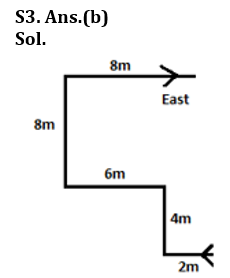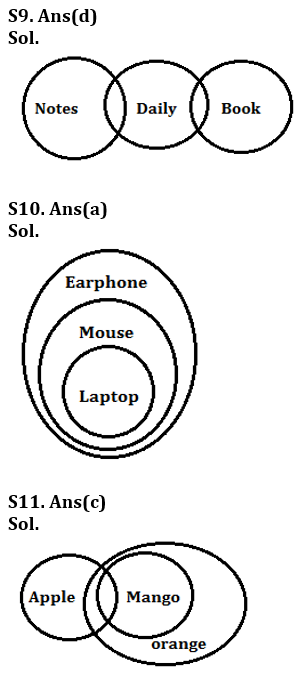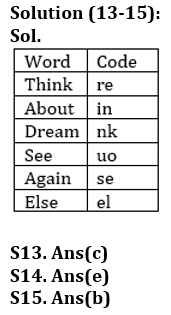Latest Banking jobs   »

# Reasoning Ability Quiz For IDBI AM/ Bank of India PO 2023 -18th March

Q1. In a class in which all are facing north A’s position is 21st from the right and B’s position is 21st from the left but C who is two places to the left of A is sitting on the immediate right of B, then how many students are there in the class?
(a) 45
(b) 39
(c) 42
(d) 44
(e) 46

Q2. A person starts from point A, walks 10 m in south direction. Then he takes two consecutive left turns and walked 8m and 16m respectively. Then takes two consecutive right turns and walks 4 m and10 m respectively. What is the shortest distance from point A to the final point?
(a) 20 m
(b) 3√10 m
(c) 4√10 m
(d) 4√5 m
(e) 10 m

Q3.A walks for 2m then turns to his right. He then walks for 4m and takes a left turn. He walks for 6m and took a right turn. He walks for 8m then turns right again. After walking for 8m he finally stops facing East. In which direction did A start walking initially?
(a) North
(b) West
(c) East
(d) South
(e) Cannot be determined

Q4. If 4th, 5th, 7th and 8th letter of the word “VEGETARIAN” is used to form a meaningful word, then which among the following will be the 4th letter from the left of the so formed word. If no such word is formed then mark answer as X, if more than one word is formed then mark your answer as Z.
(a) X
(b) E
(c) Z
(d) T
(e) R

Q5. How many letter/s will remain at the same position in the word ‘LAYOUT’ when they are arranged in the alphabetical order from left to right?
(a) None
(b) One
(c) Two
(d) Three
(e) None of these

Direction (6-8): In these questions, relationship between different elements is show in the statements. The statements are followed by conclusions. Study the conclusions based on the given statements and select the appropriate answer:
(a) If only conclusion I follows.
(b) If only conclusion II follows.
(c) If either conclusion I or II follows.
(d) If neither conclusion I nor II follows.
(e) If both conclusions I and II follow.

Q6. Statements: A = G ≥ T > I > W ≤ O ≥ B, Y ≥ T > U
Conclusions: I. Y > W II. O > A

Q7. Statements: A ≤ Q = Z = M ≤ B ≥ P = Y ≤ F
Conclusions: I. B > A II. B=A

Q8. Statements: Q < G > J = Z ≤ L > M = V ≤ I > P
Conclusions: I. I ≥ J II. J > I

Direction (9-11): In each of the questions below some statements are given followed by two conclusions. You have to take the given statements to be true even if they seem to be at variance with commonly known facts. Read all the conclusions and then decide which of the given conclusions logically follows from the given statements disregarding commonly known facts.
(a) If only conclusion I follows.
(b) If only conclusion II follows.
(c) If either conclusion I or II follows.
(d) If neither conclusion I nor II follows.
(e) If both conclusions I and II follow.

Q9. Statements: Some Notes are Daily.
Some Daily are Book
Conclusion I: No Notes is book
II: All Book are Notes

Q10. Statements: All laptop are Mouse.
All Mouse are Earphone.
Conclusion I: Some Mouse are laptop
II: All Earphone are laptop

Q11. Statements: Some Apple are Mango.
All Mango are Orange
Conclusion I: Some Apple are not Orange
II: All Apple are Orange

Q12. How many pairs of letters are there in the word ‘EXAMINATION’, each of which have as many letters between then in the word as they have between then in the English alphabet?
(a) Two
(b) Three
(c) Four
(d) One
(e) None of these

Directions (13-15): Study the information carefully and answer the questions given below.
In the coded language,
“Think About Dream” is coded as “nk in re”
“See Again About” is coded as “se in uo”
“Dream See Else” is coded as “nk uo el”

Q13. What is the code for the word “Again”?
(a) in
(b) nk
(c) se
(d) uo
(e) None of these

Q14. What is the code for the word “Dream Else”?
(a) re uo
(b) uo in
(c) nk se
(d) re nk
(e) None of these

Q15. What is the word for the code “uo”?
(a) Else
(b) See
(c) Think
(d) Again
(e) Either (a) or (d)

Solutions

S1. Ans.(d)
S2. Ans.(c)S4. Ans.(c)
S5. Ans.(b)

Solution (6-8):
S6. Ans(a)
Sol. I. Y > W(True) II. O > A(False)

S7. Ans(c)
Sol. I. B > A(False) II. B=A(False)

S8. Ans(c)
Sol. I. I ≥ J(False) II. J > I(False)S12. Ans(a)
Sol. EI, NO## FAQs

### What is the selection process of the IDBI AM?

The selection process of IDBI AM is Online exam and Interview.

#### Congratulations!Union Budget 2023-24: Free PDF